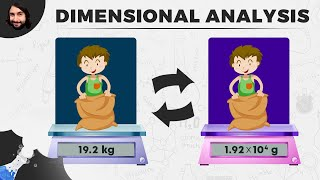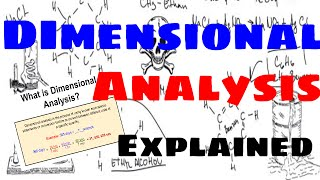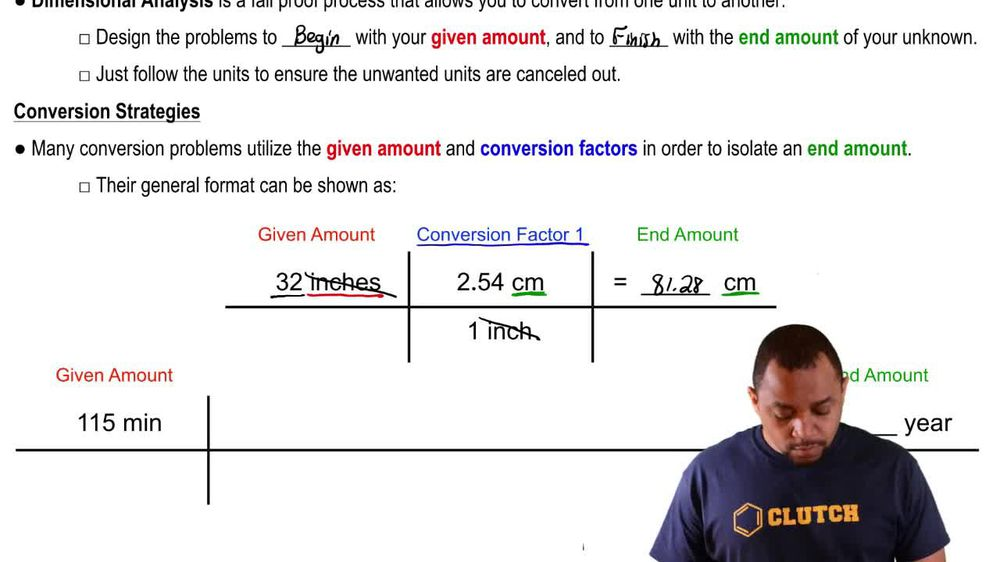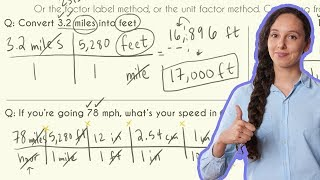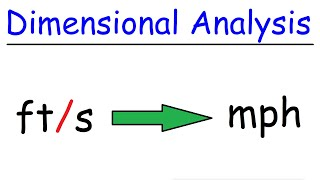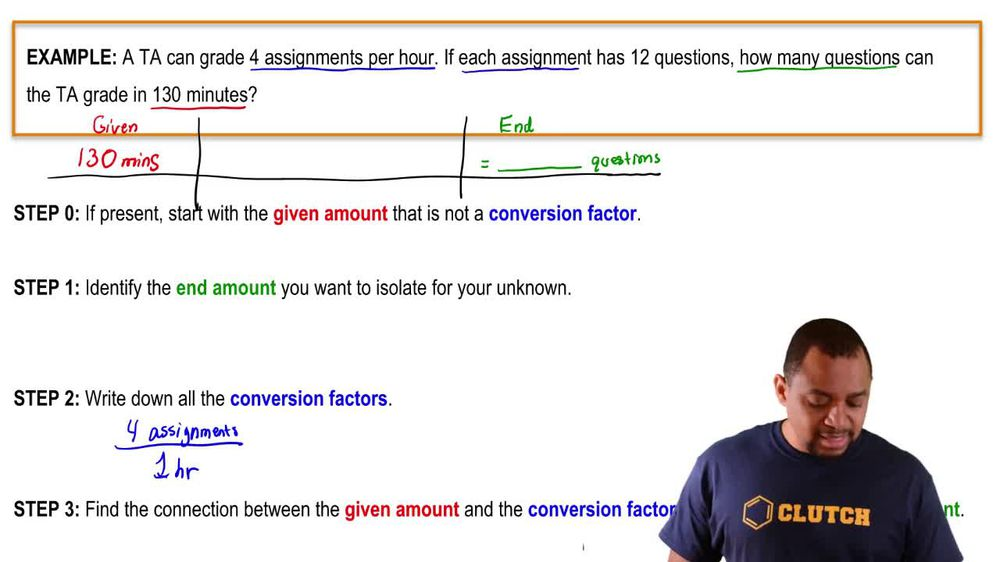Start typing, then use the up and down arrows to select an option from the list.
1. 1. Intro to General Chemistry2. Dimensional Analysis
Problem

# (c) If an automobile is able to travel 400 km on 47.3 L of gasoline, what is the gas mileage in miles per gallon?

Relevant Solution1m
Play a video:
Hi everyone today, we have a question asking us to calculate the gas rating in ounces per yard of a vehicle that consumes 0.160 decimal tres cubed per meter. Our first step here is going to be able to remember our conversion factors So we know that one m Equals 1.094 yards and we know that one decimate. Our cubed equals .814oz. And now we're going to put this into an equation. So we're going to start with our 0. decimate as cube per meter. And we're going to multiply that by a conversion factor. So 33 . ounces Over one decimate er cubed. And that's going to cancel out our Destiny meters cubed. And then we're going to multiply that by one m Over 1.094 yards and that is going to equal 0.495 ounces per yard. And that is our final answer. Thank you for watching. Bye.